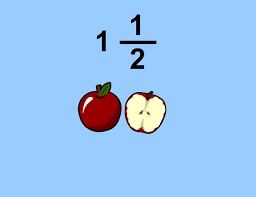# What is 29

What is the product of negative 2 and 2 over 5 and negative 3 and 5 over 6? Enter your answer as a mixed number or improper fraction in the simplest form.

x =  9 15 = 46/5

### Step-by-step explanation:Did you find an error or inaccuracy? Feel free to write us. Thank you!

Tips for related online calculators
Need help calculating sum, simplifying, or multiplying fractions? Try our fraction calculator.
Need help with mixed numbers? Try our mixed-number calculator.